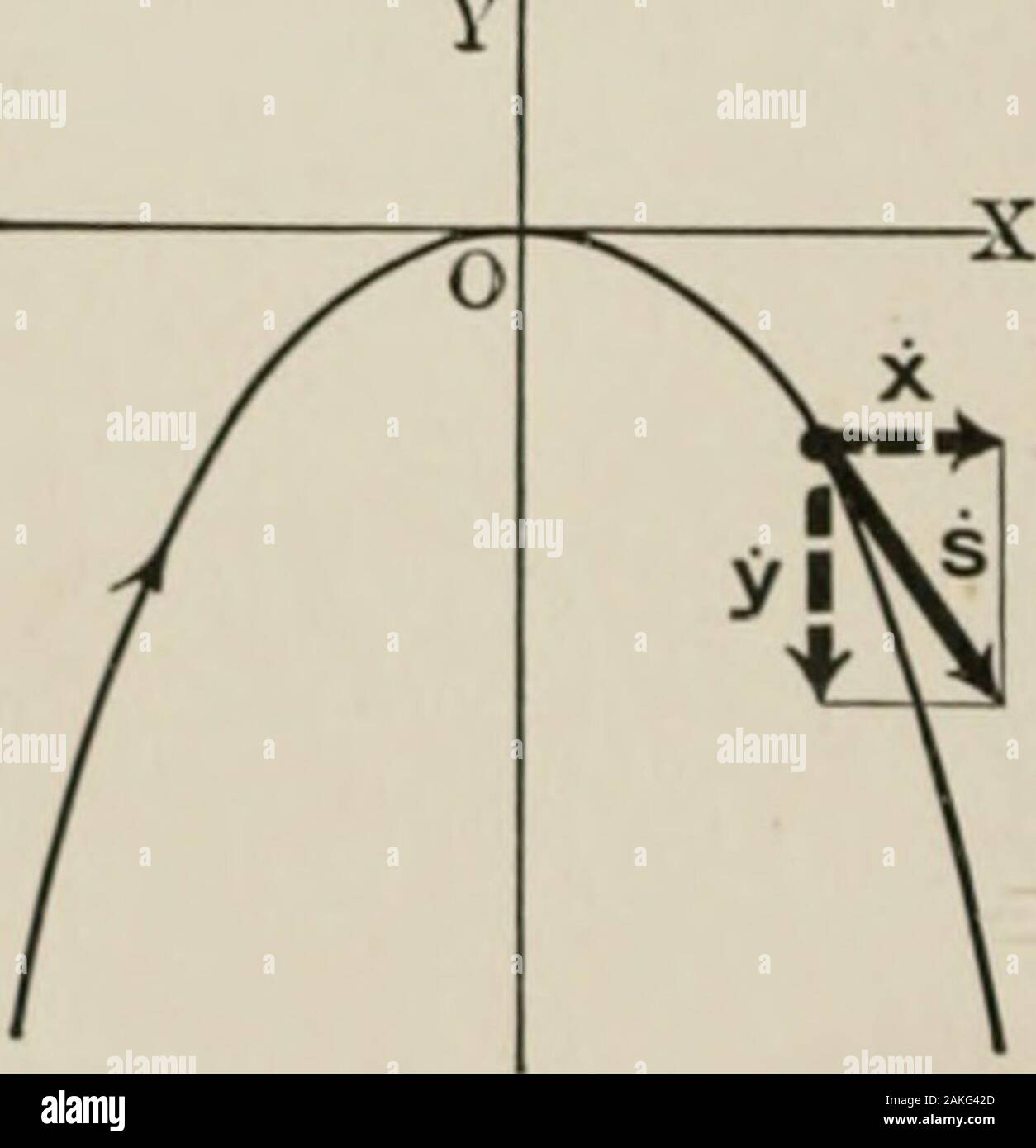# Analytical mechanics for students of physics and engineering . When, as in the case of Fig. 48, the particle moves in thejcy-plane, z = 0, therefore The direction of v, in this case, is given by tan0 = H-,x where 0 is the angle v makes with the x-axis. (HI) (IV) ILLUSTRATIVE EXAMPLE. Find the path, the velocity, and the components of the velocity of a,particle which moves so that its position at any instant is given by thefollowing equations: x = at, (a) y=-hgt*. (b) Eliminating t between (a) and (b), we obtain 2a2 for the equation of the path, therefore the path is a parabola, Fig. 49. To fin## Image details

Contributor:

The Reading Room / Alamy Stock Photo

Image ID:

2AKG42D

File size:

Releases:

Model - no | Property - noDo I need a release?

Dimensions:

1553 x 1609 px | 26.3 x 27.2 cm | 10.4 x 10.7 inches | 150dpi